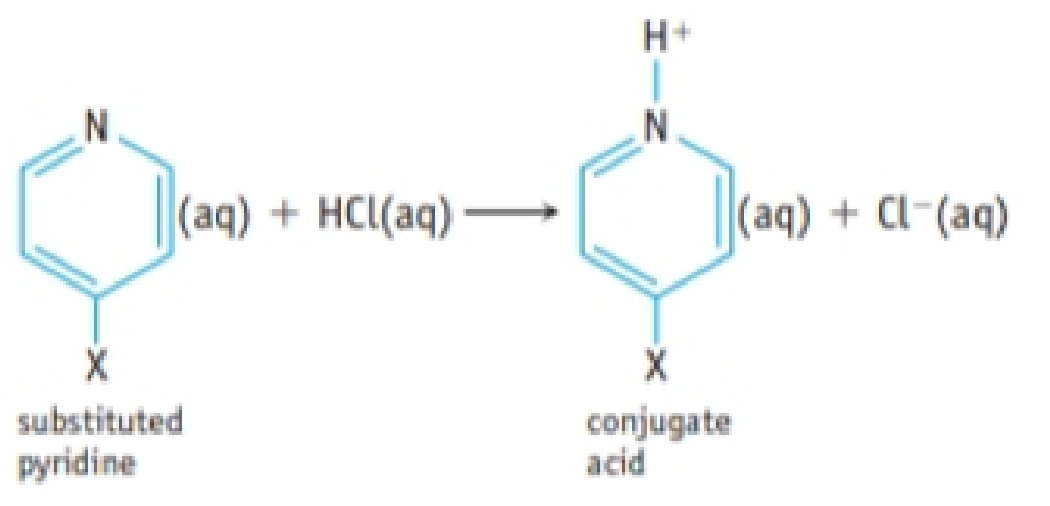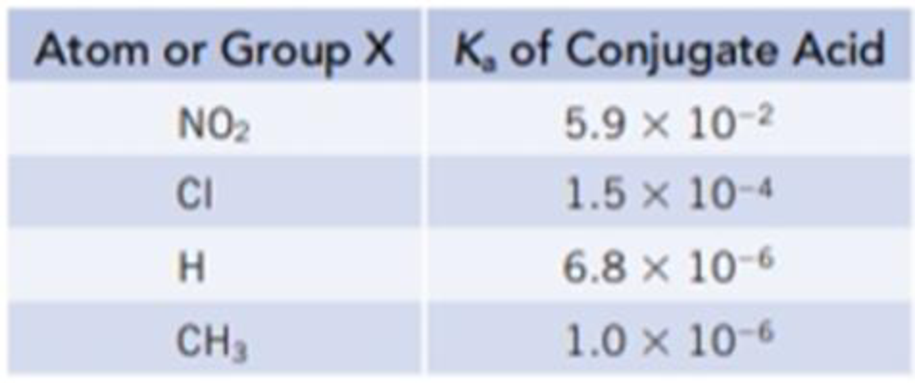# A hydrogen atom in the organic base pyridine, C 5 H 5 N, can be substituted by various atoms or groups to give XC 5 H 4 N, where X is an atom such as Cl or a group such as CH 3 . The following table gives K a values for the conjugate acids of a variety of substituted pyridines. (a) Suppose each conjugate acid is dissolved in sufficient water to give a 0.050 M solution. Which solution would have the highest pH? The lowest pH? (b) Which of the substituted pyridines is the strongest Brønsted base? Which is the weakest Brønsted base?### Chemistry & Chemical Reactivity

9th Edition
John C. Kotz + 3 others
Publisher: Cengage Learning
ISBN: 9781133949640

#### Solutions

Chapter
Section### Chemistry & Chemical Reactivity

9th Edition
John C. Kotz + 3 others
Publisher: Cengage Learning
ISBN: 9781133949640
Chapter 16, Problem 112IL
Textbook Problem
218 views

## A hydrogen atom in the organic base pyridine, C5H5N, can be substituted by various atoms or groups to give XC5H4N, where X is an atom such as Cl or a group such as CH3. The following table gives Ka values for the conjugate acids of a variety of substituted pyridines.(a) Suppose each conjugate acid is dissolved in sufficient water to give a 0.050 M solution. Which solution would have the highest pH? The lowest pH? (b) Which of the substituted pyridines is the strongest Brønsted base? Which is the weakest Brønsted base?

(a)

Interpretation Introduction

Interpretation:

The conjugate acid which have the highest pH and which have the lowest pH has to be stated.

Concept Introduction:

The pH is a measure of Hydronium ion concentration. The expression for pH is,

pH=log[H3O+] (1)

Higher the value of Ka, stronger the acid and for an acidic solution pH value is always less than 7. That means higher the value of Ka for a stronger acid smaller the value of pH.

### Explanation of Solution

An equilibrium constant (K) is the ratio of concentration of products and reactants raised to appropriate stoichiometric coefficient at equlibrium.

For Any acid HA,

HA(aq)+H2O(l)H3O+(aq)+A(aq)

The relative strength of an acid and base in water can be also expressed quantitatively with an equilibrium constant as follows:

Ka=[H3O+][A][HA] (2)

An equilibrium constant (K) with subscript a indicate that it is an equilibrium constant of an acid in water.

Given:

The Ka value for each conjugate acids are:

Group(X)KaofAcid[4X(C5H5N+)]NO25.9×102Cl1.5×104H6.8×106CH31×106

Initial Concentration of each solution is 0.05M.

Set up an ICE table for the reaction of 4X(C5H5N+) with water at equilibrium,

Equilibrium4X(C5H5N+)(aq)+H2O(l)4X(C5H4N)(aq)+H3O+(aq)Initial(M)0.0500Changex+x+xAtEquilibrium0.05xxx

Substitute the values in equation (2) to calculate x for each acid,

Ka=x20.05x (3)

Substitute the value of Ka for 4NO2(C5H5N+) in equation (3) to calculate the value of x,

5.9×102=x20.05xx=0.054

The value of x is equal to the concentration of hydronium ion that is 0.054 molL1.

Substitute the value of hydronium ion concentration in equation (1) to calculate the value of pH,

pH=log(0.054)=1.26

Therefore, the value of pH for 4NO2(C5H5N+) is 1

(b)

Interpretation Introduction

Interpretation:

Strongest bronsted base and weakest bronsted base is to be stated in the given substituted pyridines.

Concept Introduction:

A conjugate acid-base pair contains two compounds that differ only by a hydrogen ion and a charge of +1. Thus, 4X(C5H5N+) and 4X(C5H4N) are conjugate acid-base pair. In this pair, 4X(C5H4N) is a conjugate base of an acid 4X(C5H5N+).

The stronger the acid, the weaker its conjugate base and vice-verca. That is, the larger the values of Ka, the smaller the values of Kb.

### Still sussing out bartleby?

Check out a sample textbook solution.

See a sample solution

#### The Solution to Your Study Problems

Bartleby provides explanations to thousands of textbook problems written by our experts, many with advanced degrees!

Get Started

Find more solutions based on key concepts
The nutrition objectives for the nation, as part of Healthy People 2020, a. envision a society in which all peo...

Nutrition: Concepts and Controversies - Standalone book (MindTap Course List)

Even a small loss of body weight can improve OA.

Nutrition Through The Life Cycle

What are some functions of skin?

Human Biology (MindTap Course List)

How can errors in the cell cycle lead to cancer in humans?

Human Heredity: Principles and Issues (MindTap Course List)

Match the term listed in Column A with its definition from Column B

Nutrition Through the Life Cycle (MindTap Course List)

Figure CQ10.15 shows a side view of a childs tricycle with rubber tires on a horizontal concrete sidewalk. If a...

Physics for Scientists and Engineers, Technology Update (No access codes included)

Why is water a polar molecule (review Chapter 6)?

Oceanography: An Invitation To Marine Science, Loose-leaf Versin